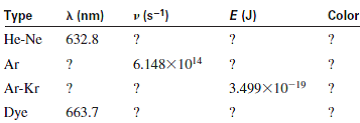Problem

A laser (light amplification by stimulated emission of radiation) provides nearly monoc...

A laser (light amplification by stimulated emission of radiation) provides nearly monochromatic high-intensity light. Lasers are used in eye surgery, CD/DVD players, basic research, and many other areas. Some dye lasers can be “tuned” to emit a desired wavelength. Fill in the blanks in the following table of the properties of some common lasers:Step-by-Step Solution

Solution 1

For He-Ne laser:

Given -

The wave length of the light $$=632.8 \mathrm{~nm}$$

Since, frequency, $$v=\frac{c}{\lambda}$$

Where, $$c=3.0 \times 10^{8} \mathrm{~m} / \mathrm{s}$$ (velocity of the light)

Therefore, frequency corresponds to the given wave length, $$v=\frac{3.0 \times 10^{8} \mathrm{~m} / \mathrm{s}}{632.8 \mathrm{~nm} \times \frac{10^{-9} \mathrm{~m}}{1 \mathrm{~nm}}}$$ $$=4.74 \times 10^{14} / \mathrm{s}$$

From the relation between the energy and frequency, $$E=h v$$

Energy of the photon in the He-Ne laser lamp, $$E=6.626 \times 10^{-34} \mathrm{~J} . \mathrm{s} \times 4.74 \times 10^{14} / \mathrm{s}$$ $$=3.14 \times 10^{-19} \mathrm{~J}$$

The wave length at $$632.8 \mathrm{~nm}$$ indicates that it has the pale orange color.

For Ar laser:

Given -

The frequency of the light given, $$v=6.148 \times 10^{14}$$

Since, frequency, $$v=\frac{c}{\lambda}$$

Where, $$c=3.0 \times 10^{8} \mathrm{~m} / \mathrm{s}$$ (velocity of the light)

$$\therefore$$ Wave length, $$\lambda=\frac{3.0 \times 10^{8} \mathrm{~m} / \mathrm{s}}{6.148 \times 10^{14} / \mathrm{s}}$$ $$=487.9 \times 10^{-9} \mathrm{~m}$$ $$=487.9 \mathrm{~nm} \quad\left[\because 1 \mathrm{~nm}=10^{-9} \mathrm{~m}\right]$$

From the relation between the energy and frequency, $$E=h v$$

Energy of the photon in the He-Ne laser lamp, $$E=6.626 \times 10^{-34} \mathrm{~J} . \mathrm{s} \times 6.148 \times 10^{14} / \mathrm{s}$$ $$=4.07 \times 10^{-19} \mathrm{~J}$$

The wave length at $$487.9 \mathrm{~nm}$$ indicates that it has the blue color.

For Ar-Kr laser:

Given -

Energy of the photon, $$E=3.499 \times 10^{-19} \mathrm{~J}$$

From the relation between the energy and frequency, $$E=h v$$

Therefore, Frequency of the light, $$v=\frac{E}{h}$$

\begin{aligned} &=\frac{3.499 \times 10^{-19} \mathrm{~J}}{6.626 \times 10^{-34} \mathrm{~J} . \mathrm{s}} \\ &=5.280 \times 10^{14} / \mathrm{s} \end{aligned}

Similarly the wave length of the light, $$\lambda=\frac{c}{v}$$

\begin{aligned} &=\frac{3.0 \times 10^{8} \mathrm{~m} / \mathrm{s}}{5.280 \times 10^{14} / \mathrm{s}} \\ &=568 \times 10^{-9} \mathrm{~m} \\ &=568 \mathrm{~nm}\left[\because 1 \mathrm{~nm}=10^{-9} \mathrm{~m}\right] \end{aligned}

The wave length at $$568 \mathrm{~nm}$$ indicates the color light yellow color.

For Dye laser:

Given -

The wave length of the light $$=663.7 \mathrm{~nm}$$

Since, frequency, $$v=\frac{c}{\lambda}$$

Where, $$c=3.0 \times 10^{8} \mathrm{~m} / \mathrm{s}$$ (velocity of the light)

Therefore, frequency corresponds to the given wave length, $$\quad v=\frac{3.0 \times 10^{8} \mathrm{~m} / \mathrm{s}}{663.7 \mathrm{~nm} \times \frac{10^{-9} \mathrm{~m}}{1 \mathrm{~nm}}}$$ $$=4.52 \times 10^{14} / \mathrm{s}$$

From the relation between the energy and frequency, $$E=h v$$

Energy of the photon in the He-Ne laser lamp, $$E=6.626 \times 10^{-34} \mathrm{~J} . \mathrm{s} \times 4.52 \times 10^{14} / \mathrm{s}$$ $$=3.0 \times 10^{-19} \mathrm{~J}$$

The wave length at $$663.7 \mathrm{~nm}$$ indicates that it has the dark orange color.

Type lambda(nm) v(s^(-1)) E(J) Color
He-Ne 632.8 4.74 xx10^(14) s^(-1) 3.14 xx10^(-19) J Pale
Ar 487.9 6.148 xx10^(14) s^(-1) 4.07 xx10^(-19) J Blue
Ar-Kr 568 5.280 xx10^(14)//s 3.499 xx10^(-19) J Yellow
Dye 663.7 4.52 xx10^(14)//s 3.0 xx10^(-19) J Dark orange